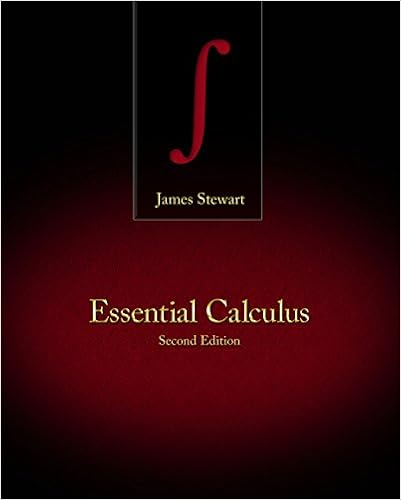# Lastly for the case n 0 we will use complex

• Test Prep
• 434
• 100% (1) 1 out of 1 people found this document helpful

This preview shows page 347 - 352 out of 434 pages.

##### We have textbook solutions for you!
The document you are viewing contains questions related to this textbook.The document you are viewing contains questions related to this textbook.
Chapter 10 / Exercise 51
Essential Calculus
StewartExpert Verified
Induction.Lastly, for the casen <0 we will use complex arithmetic. Sincen <0,n=-mfor somemN.(cosθ+isinθ)n= (cosθ+isinθ)-m=1(cosθ+isinθ)m=1(cos+isin)= cos-isin= cos(-) +i(sin(-))= cos+isin
##### We have textbook solutions for you!
The document you are viewing contains questions related to this textbook.The document you are viewing contains questions related to this textbook.
Chapter 10 / Exercise 51
Essential Calculus
StewartExpert Verified
348Chapter 40De Moivre’s TheoremCorollary 2Ifz=r(cosθ+isinθ) andnis an integer,zn=rn(cos+isin)40.3Complex ExponentialsIf you were asked to find a real-valued functionywith the property thatdydx=kyandy= 1 whenx= 0for some constantk, you would choosey=ekxAnd if you were asked to find the derivative off(θ) = cosθ+isinθwhereiwas treated asany other constant you would almost certainly writedf(θ)=-sinθ+icosθbut thendf(θ)=-sinθ+icosθ=i(cosθ+isinθ) =if(θ)and sodf(θ)=if(θ) andf(θ) = 1 whenθ= 0Definition 40.3.1ComplexExponentialBy analogy, we define thecomplex exponential functionbye= cosθ+isinθAs an exercise, prove the following.Proposition 3(Properties of Complex Exponentials (PCE))e·e=ei(θ+φ)en=einθnZThe polar form of a complex numberzcan now be written asz=rewherer=|z|andθis an argument ofz.
Section 40.5More Examples349Out of this arises one of the most stunning formulas in mathematics. Settingr= 1 andθ=πwe gete= cosπ+isinπ=-1 + 0i=-1That ise+ 1 = 0Who would have believed thate, i, π,1 and 0 would have such a wonderful connection!40.4More Examples1. This question asks you to compute (3 +i)4in two ways.Write your answer instandard form.(a) Use the Binomial Theorem.(b) Use De Moivre’s Theorem.Solution:(a) Using the Binomial Theorem we have(3 +y)4=4Xr=04r(3)4-rir=40(3)4-0i0+41(3)4-1i1+42(3)4-2i2+43(3)4-3i3+44(3)4-4i4= 9 + 4·33i-6·3-4i3 + 1=-8 + 83i(b) Using De Moivre’s Theorem we have First, write3 +iin polar form. The modulusisr=q32+ 12= 2. An argument isπ6. Thus,3 +i= 2(cosπ6+isinπ6). By DeMoivre’s Theorem2cosπ6+isinπ64= 24cos4π6+isin4π6= 16cos2π3+isin2π3= 16-12+3i2!=-8 + 83i(c) Writez=ei5π/123-6in the formx+iywithx, yR.Solution:z=ei5π/123-6= (3)6(ei5π/12)-6= 33e-i5π/2= 27(e-iπ/2) =-27i.
350Chapter 40De Moivre’s Theorem40.5Practice1. Compute each of the following twice:once using the Binomial Theorem and onceusing De Moivre’s Theorem. Write your answer in standard form.(a) (3-3i)4(b) (2-23i)4
Chapter 41Roots of Complex Numbers41.1ObjectivesThe content objectives are:1. State and prove theComplexn-th Roots Theoremand do examples.41.2Complexn-th RootsDefinition 41.2.1Complex RootsIfais a complex number, then the complex numbers that solvezn=aare called thecomplexn-th roots. De Moivre’s Theorem gives us a straightforward wayto find complexn-th roots ofa.Theorem 1(Complexn-th Roots Theorem (CNRT))Ifr(cosθ+isinθ) is the polar form of a complex numbera, then the solutions tozn=aarenrcosθ+ 2n+isinθ+ 2nfork= 0,1,2, . . . , n-1The modulusnris the unique non-negativen-th root ofr. This theorem asserts that anycomplex number, including the reals, has exactlyndifferent complexn-th roots.
•••